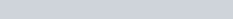# Geometric Distribution: Definition, Properties and Applications

A geometric distribution is a discrete probability distribution that illustrates the probability that a Bernoulli trial will result in multiple failures before success. A Bernoulli trial is an experiment that can have only two possible outcomes, i.e., success or failure. In a geometric distribution, a Bernoulli trial is essentially repeated until success is attained.

It is widely used in several real-life activities. For example, in the financial industry, it is used to do a cost-benefit analysis to estimate the financial benefits of making a certain decision.

### Definition of Geometric distribution

A discrete random variable X is said to have a geometric distribution if its probability density function is defined as,where p is the only parameter of of geometric distribution which satisfy  0<=p<=1 and p+q=1.

### Assumptions

There are three main assumptions.

• The trials must be independent.
• Each experiment can only result in one of two outcomes: success or failure.
• For each trial, the probability of success is denoted by p.

### Properties of Geometric Distribution

• Geometric distribution follows the lack memory property.
• The mean of geometric distribution is.
• The variance of geometric distribution is.
• Moment generating function of geometric distribution is.
• The mean of geometric distribution is smaller then its variance, since q/p2 > q/p.

### Application of Geometric distribution

• The Geometric distribution is used in Markov chain models.
• It is used in meteorological modes of weather cycles and precipitation amounts.
• The geometric distribution is often referred to as the failure time distribution.
• It would be used to describe the number of interviews that have to be conducted by a selection board to appoint the first acceptable candidate.